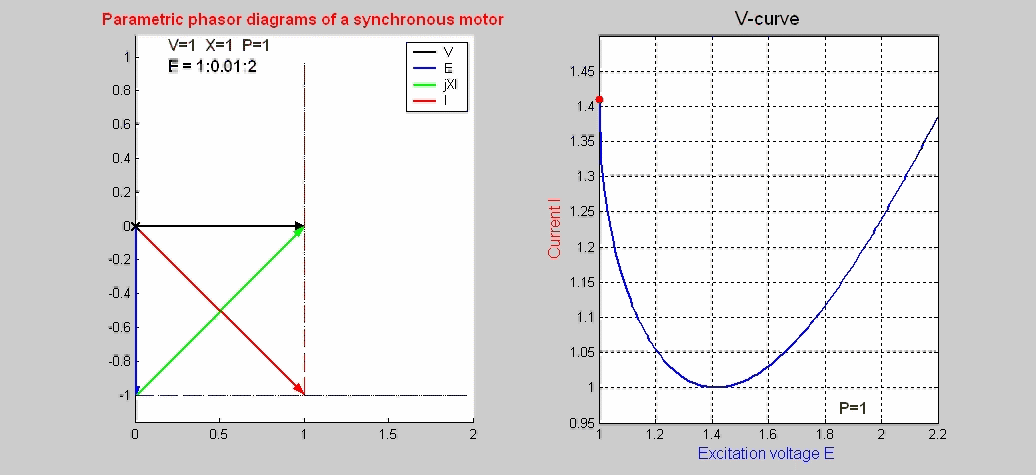﻿ V curves of a synchronous motor

SYNCHRONOUS MOTOR CHARACTERISTICS (V-curves )

The steady-state characteristics of a synchronous motor represented by phasor diagrams are shown as function of the excitation voltage E: for low values of E, the motor is said to be under excited and the current I lags the terminal voltage V where as, for large values of E, the motor becomes over excited with the current now leading the voltage.  Note that the locus of the current phasor is a vertical line meaning that I cos(φ) is constant; similarly  the locus of E is a horizontal line satisfying the condition that E sin(δ) is constant; both constraints are the consequence of maintaining constant power P = I V cos(φ) = E V sin(δ) / X.  The basic phasor equation is V = E + jX I. The associated V-curve (I versus E) is also plotted.